# Category Archives: Math Foundations

[This is the 4th and last installment in the current series on mini-golf and ellipse geometry. See the previous ones here: #1, #2, #3.]

We must settle one more question to round out our elliptical arc: Why does light, when shot from one focus of an ellipse-shaped mirrored room, reflect back to the other focus? To answer this question, we’ll need a Fact, a Formalism, and a Fairy Tale.

### The Fact

Recall that, in the previous post, we saw that ellipses can be described by distances: Any ellipse has two focus points F1 and F2 so that the total length of broken path F1XF2 is the same for every point X on the ellipse; let d be this common total distance. In fact, more is true: the length of F1XF2 is smaller than d if X is inside the ellipse, and larger than d if X is outside.Left: The distance of the broken path is greater, equal, or less than d depending on whether the central vertex is outside, on, or inside the ellipse. Right: A proof of the “outside” case.

To prove this fairly intuitive fact, we’ll use the “straight line principle”: the shortest distance between two points is a straight line. Indeed, when X is outside the ellipse (see right diagram above), straight-line path YF2 is shorter than the path YXF2 that detours through X, and so d = F_1 Y F_2 < F_1 Y X F_2[/latex]. See if you can fill in the case where X is inside the ellipse.

### The Formalism

Recall that when light bounces off a straight mirror, the angle of incidence equals the angle of reflection. But here we’re discussing light bouncing off an ellipse, which is decidedly not straight. So we need to formally describe how light reflects off curved mirrors.

If we zoom into where the incoming light ray strikes a curved mirror (illustrated above), the mirror closely resembles a straight line, specifically its tangent line. This suggests that the light should behave as if it is reflecting off of this line, with equal angles as marked. This is indeed the rule governing ideal reflections on curved mirrors: the angles of incidence and reflection, as measured from the tangent line, should be equal.

### The Fairy Tale

The last ingredient involves Little Red Riding Hood and her thirsty grandmother. Red is delivering cake and wine from her mother (point M) to her grandmother (point G), but she must first fill a bucket of water at the nearby stream S, which is conveniently shaped like a straight line. She was warned by her Brothers to watch out for a big bad wolf, so she must minimize her total walking distance. Where on the stream should she fill her bucket to minimize this distance?Left: Paths from M to S to G transform into paths from M’ to G, the shortest of which is straight. Right: This straight path behaves like a mirror.

To answer this, imagine reflecting the first leg of Red’s journey across line S, so her path from M to S to G gets reflected to a path from M’ to G. The reverse may be done as well: any path from M’ to G turns into a path from M to G that stops somewhere along S. So we just need to find the shortest path from M’ to G. But this is easy: it’s just the straight path M’G. So Red’s shortest path from M to S to G is the one that stops at Z.

Notice that this shortest path is the one with equal angles as marked. This means Red’s best strategy is to pretend the stream is a mirror and to follow the light ray that bounces directly to grandma’s house. This neatly exemplifies Fermat’s principle, which says that light tends to follow the fastest routes.

### The Proof

With these pieces in place, we can finish today’s question in a flash. Let’s say light from focus F1 hits an ellipse at point X, as illustrated below. Why does this ray bounce off the ellipse toward F2? If we draw the tangent line L at point X, by The Formalism above, this question is equivalent to: why does the light ray bounce off of line L toward F2?Why are the two marked angles equal? Because the white path solves Red’s Fairy Tale problem.

Let’s reimagine this Grimm scenario by thinking of F1 as Red’s mother’s house, F2 as grandma’s house, and L as the stream. I claim F1XF2 is the shortest path for Red to take. Why? If Y is any other point on line L, then Y is outside the ellipse, so by The Fact above, F1YF2 has distance longer than d. So F1XF2 is indeed the shortest. But by The Fairy Tale, we know that this shortest route behaves like light bouncing off of line L, i.e., the marked angles are indeed equal. So we’re done!

# What Makes Ellipses… Ellipses?

Last time we used wild properties of ellipses to build some really easy—and some really devilish—golf courses. Specifically, I claimed that every ellipse has two magical points F1 and F2 (called foci) such that a ray from F1 always bounces off the ellipse and lands precisely at F2, and furthermore, this path always has the same length. Why does this happen? And how do we find these foci?

Let’s focus(!) on the last question first. Recall that an ellipse is a stretched circle. In other words, an ellipse is what forms when you slice a tall, circular tube (cylinder) along a slant: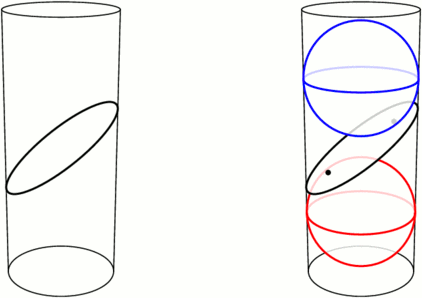Left: An ellipse is formed by slicing a cylinder. Right: Fitting spheres above and below the cut locates the ellipse’s foci.

Take a sphere that snugly fits inside the tube, and drop it down until it touches the ellipse-slice at a single point F1. Do the same with a sphere underneath, touching the slice at F2. These points turn out to be the foci of the ellipse. Let’s see why.

We can use this tubular setup to answer one mystery from earlier: for any point X on the ellipse, the sum of distances of XF1 and XF2 is always the same! The proof lies in the following animation. Segments XF1 and XA have the same length because they’re both tangent to the upper sphere from X, and similarly, XF2=XB. So the sum XF1+XF2 is just the length of segment AB, the height between the spheres’ equators.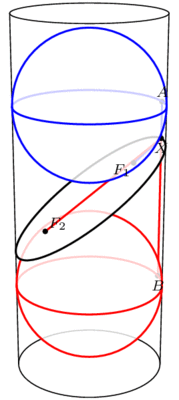The sum F1X+XF2 equals the length of AB and therefore does not change when X moves.

This has two neat consequences. First, it provides an elementary method for drawing ellipses (in real life!): all you need are two push pins and a loop of string, as illustrated below. The string ensures that the sum XF1+XF2 stays fixed while you trace the pen around, as long as you’re careful to keep the string taut throughout.

Second, what happens if we slice a cone instead of a cylinder? Perhaps surprisingly, we still get an ellipse! Indeed, as above, we can create a sphere on either side of the slice that snugly fits against the slice and the walls of the cone (the so-called Dandelin spheres), and exactly the same proof shows that XF1+XF2 stays constant as X moves around the edge.

But wait, there’s still an unanswered question! We’ve seen that the path F1X+XF2 has a fixed length, but why does light bounce off an ellipse along such a path? This is what we really cared about for mini-golf! Come back next time for the answer, and in the meantime, have a great 2 weeks.

# Logic Under Construction

In last week’s discussion of proofs by contradiction and nonconstructive proofs, we showed:

Theorem: There exist irrational numbers $$x$$ and $$y$$ with the property that $$x^y$$ is rational.

However, our proof was nonconstructive: it did not pinpoint explicit values for $$x$$ and $$y$$ that satisfy the condition, instead proving only that such numbers must exist. Would a more constructive proof be more satisfying? Let’s see! I claim $$x=\sqrt{2}$$ and $$y=\log_2 9$$ work, because $$\sqrt{2}$$ we already know to be irrational, $$y=\log_2 9$$ can be similarly proved to be irrational (try this!), and $$x^y = \sqrt{2}^{\log_2 9} = \sqrt{2}^{\log_{\sqrt{2}}3}=3,$$ which is rational.

Let’s further discuss why last week’s proof was less satisfying. The following rephrasing of this proof may help shed some light on the situation:

Proof: Assume the theorem were false, so that any time $$x$$ and $$y$$ were irrational, $$x^y$$ would also be irrational. This would imply that $$\sqrt{2}^{\sqrt{2}}$$ would be irrational, and by applying our assumption again, $$\left(\sqrt{2}^{\sqrt{2}}\right)^{\sqrt{2}}$$ would also be irrational. But this last number equals 2, which is rational. This contradiction disproves our assumption and thereby proves the theorem, QED.

So perhaps this argument seems less satisfactory simply because it is, at its core, a proof by contradiction. It does not give us evidence for the positive statement “$$x$$ and $$y$$ exist”, but instead only for the negative statement “$$x$$ and $$y$$ don’t not exist.” (Note the double negative.) This distinction is subtle, but a similar phenomenon can be found in the English language: the double negative “not bad” does not mean “good” but instead occupies a hazy middle-ground between the two extremes. And even though we don’t usually think of such a middle-ground existing between logic’s “true” and “false”, proofs by contradiction fit naturally into this haze. In fact, these ideas motivate a whole branch of mathematical logic called Constructive logic that disallows double negatives and proofs by contradiction, instead requiring concrete, constructive justifications for all statements.

But wait; last week’s proof that $$\sqrt{2}$$ is irrational used contradiction, and therefore is not acceptable in constructive logic. Can we prove this statement constructively? We must show that $$\sqrt{2}$$ is not equal to any rational number; what does it even mean to do this constructively? First, we turn it into a positive statement: we must show that $$\sqrt{2}$$ is unequal to every rational number. And how do we constructively prove that two numbers are unequal? By showing that they are measurably far apart. So, here is a sketch of a constructive proof: $$\sqrt{2}$$ is unequal to every rational number $$a/b$$ because $$\left|\sqrt{2} – \frac{a}{b}\right| \ge \frac{1}{3b^2}.$$ See if you can verify this inequality!

PS. In case you are still wondering whether $$\sqrt{2}^{\sqrt{2}}$$ is rational or irrational: It is irrational (moreover, transcendental), but the only proof that I know uses a very difficult theorem of Gelfond and Schneider.

### Notes

1. This inequality would also have to be proven in a constructive manner. See these Wikipedia articles for more information: Intuitionistic logic (another name for Constructive logic) and Square root of 2: Constructive proof. []

# Methods of Irrationality

Being a mathematician requires you to think in strange ways.

For starters, you might think that mathematicians spend all day pondering purely theoretical things that only exist in Mathematical abstraction, right? Well, it can get even stranger than that: sometimes we have to think about things that don’t exist, even in the abstraction! It’s called a proof by contradiction, and here’s an example:

Theorem: The number $$\sqrt{2}$$ is irrational.

Proof: To prove that $$\sqrt{2}$$ is irrational, we have to show that we can never write it as a ratio of integers, $$\sqrt{2}=a/b$$. So let’s assume we can find such a fraction and see where it leads us.

Let’s cancel common factors in the numerator and denominator so that $$a/b$$ is in lowest terms. Squaring our equation shows that $$a^2=2b^2$$, so $$a^2$$ is even and so $$a$$ itself is even. Since $$a/b$$ is in lowest terms, $$b$$ must be odd.

Since $$a$$ is even, $$a^2$$ is in fact divisible by 4. On the other hand, since $$b$$ is odd, $$2b^2$$ is not divisible by 4. But then the integer $$a^2=2b^2$$ is both divisible by 4 and not divisible by 4, which is absurd! The only explanation for this contradiction is that our original assumption—that $$\sqrt{2}$$ is rational—is false. So we’re done with the proof! Notice that we spent most of our effort reasoning about integers that never existed (specifically $$a$$ and $$b$$).

But that’s not the only twisted thing about Mathematical thinking. Here’s a delightfully short yet aggravatingly unsatisfying proof:

Theorem: There exist irrational numbers $$x$$ and $$y$$ such that $$x^y$$ is rational.

Proof: We already know that $$\sqrt{2}$$ is irrational, so maybe we can use $$\sqrt{2}^{\sqrt{2}}$$. Is this number rational? If it is, then we’re done: $$x=\sqrt{2},y=\sqrt{2}$$ solves the problem. But what if $$\sqrt{2}^{\sqrt{2}}$$ is irrational? In this case, I claim $$x=\sqrt{2}^{\sqrt{2}},y=\sqrt{2}$$ works: indeed, $$\left(\sqrt{2}^{\sqrt{2}}\right)^{\sqrt{2}} = \sqrt{2}^{\sqrt{2} \cdot\sqrt{2}} = \sqrt{2}^2 = 2,$$ which is rational. In either case the required numbers $$x$$ and $$y$$ exist, so this completes the proof.

But wait; which is it? The proof shows us that one of the pairs $$x=\sqrt{2},y=\sqrt{2}$$ or $$x=\sqrt{2}^{\sqrt{2}},y=\sqrt{2}$$ works, but it doesn’t tell us which one! So, have we proven the theorem? Yes, technically, but only nonconstructively.

Frustrating, isn’t it?

# Spherical Surfaces and Hat Boxes

To round off our series on round objects (see the first and second posts), let’s compute the sphere’s surface area. We can compute this in the same way we related the area and circumference of a circle two weeks ago. Approximate the surface of the sphere with lots of small triangles, and connect these to the center of the sphere to create lots of triangular pyramids. Each pyramid has volume $$\frac{1}{3}(\text{area of base})(\text{height})$$, where the heights are all nearly $$r$$ and the base areas add to approximately the surface area. By using more and smaller triangles these approximations get better and better, so the volume of the sphere is $$\frac{4}{3}\pi r^3 = \frac{1}{3}(\text{surface area})\cdot r,$$ meaning the surface area is $$4\pi r^2$$. (This and previous arguments can be made precise with the modern language of integral calculus.)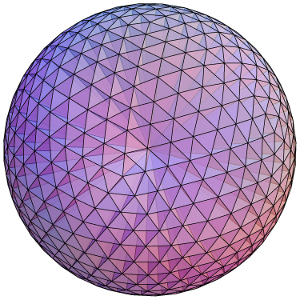Dividing a sphere into many pyramids connected to the center allows us to relate the sphere's surface area and volume.

Here’s an elegant way to rephrase this result: The surface area of a sphere is equal to the area of the curved portion of a cylinder that exactly encloses the sphere. In fact, something very surprising happens here!:

Archimedes’ Hat-Box Theorem: If we draw any two horizontal planes as shown below, then the portions of the sphere and the cylinder between the two planes have the same surface area.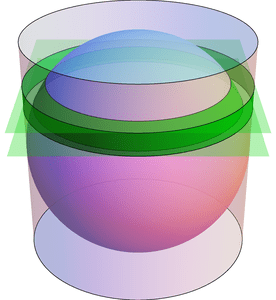Any two horizontal planes cut off a band on the sphere and another band on the enclosing cylinder. Archimedes' Hat-Box Theorem says that these bands have the same area. (The planes shown here have heights $$0.4\cdot r$$ and $$0.6\cdot r$$ above the equator.)

We can prove this with (all!) the methods in the last few posts; here’s a quick sketch. To compute the area of the “spherical band” (usually called a spherical zone), first consider the solid spherical sector formed by joining the spherical zone to the center: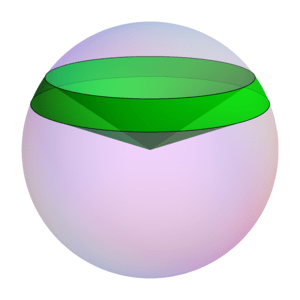The Hat-Box theorem can be proved by relating the area of the spherical zone to the volume of this spherical sector.

By dividing this into lots of triangular pyramids as we did with the sphere above, we can compute the area of the spherical zone by instead computing the sector’s volume. This volume can be computed by breaking it into three parts: two cones and the spherical segment between the two planes (on the left of the next figure). Compute the volume of the spherical segment by comparing (via Cavalieri’s Principle) to the corresponding part of the vase (from the previous post), which can be expressed with just cylinders and cones.

See if you can fill in the details!

# Slicing Spheres

Last week we saw how to compute the area of a circle from first principles. What about spheres?

To compute the volume of a sphere, let’s show that a hemisphere (with radius $$r$$) has the same volume as the vase shown in the figure below, formed by carving a cone from the circular cylinder with radius and height $$r$$. Why this shape? Here’s why: if we cut these two solids at any height $$h$$ (between 0 and $$r$$), the areas of the two slices match. Indeed, the slice—usually called cross section—of the sphere is a circle of radius $$\sqrt{r^2-h^2}$$, which has area $$\pi(r^2-h^2)$$. Similarly, the vase’s cross section is a radius $$r$$ circle with a radius $$h$$ circle cut out, so its area is $$\pi r^2-\pi h^2$$, as claimed.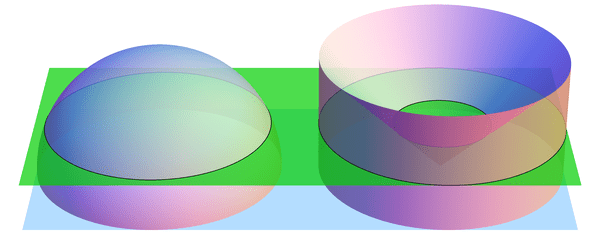When sliced by a horizontal plane at any height $$h$$, the hemisphere and vase have equal cross-sectional areas. (Shown here for $$h = 0.4\cdot r$$.) By Cavalieri's Principle, this implies that they have equal volumes.

If we imagine the hemisphere and vase as being made from lots of tiny grains of sand, then we just showed, intuitively, that the two solids have the same number of grains of sand in every layer. So there should be the same number of grains in total, i.e., the volumes should match. This intuition is exactly right:

Cavalieri’s Principle: any two shapes that have matching horizontal cross sectional areas also have the same volume.

So the volumes are indeed equal, and all that’s left is to compute the volume of the vase. But we can do this! Recall that the cone has volume $$\frac{1}{3} (\text{area of base}) (\text{height}) = \frac{1}{3}\pi r^3$$ (better yet, prove this too! Hint: use Cavalieri’s Principle again to compare to a triangular pyramid). Likewise, the cylinder has volume $$(\text{area of base}) (\text{height}) = \pi r^3$$, so the vase (and hemisphere) have volume $$\pi r^3 – \frac{1}{3} \pi r^3 = \frac{2}{3}\pi r^3$$. The volume of the whole sphere is thus $$\frac{4}{3}\pi r^3$$. Success!

The following visualization illustrates what we have shown, namely $$\text{hemisphere} + \text{cone} = \text{cylinder}.$$ The “grains of sand” in the hemisphere are being displaced horizontally by the stabbing cone, and at the end we have exactly filled the cylinder.

# Archimedes’ Circular Reasoning

Every geometry textbook has formulas for the circumference ($$C = 2 \pi r$$) and area ($$A = \pi r^2$$) of a circle. But where do these come from? How can we prove them?

Well, the first is more a definition than a theorem: the number $$\pi$$ is usually defined as the ratio of a circle’s circumference to its diameter: $$\pi = C/(2r)$$. Armed with this, we can compute the area of a circle. Archimedes’ idea (in 260 BCE) was to approximate this area by looking at regular $$n$$-sided polygons drawn inside and outside the circle, as in the diagram below. Increasing $$n$$ gives better and better approximations to the area.The n-sided regular polygons inside (blue) and outside (red) a circle can be used to approximate the area of the circle. Shown here for n=12.

Look first at the inner polygon. Its perimeter is slightly less than the circle’s circumference, $$C = 2 \pi r$$, and the height of each triangle is slightly less than $$r$$. So when reassembled as shown, the triangles form a rectangle whose area is just under $$C/2\cdot r = \pi r^2$$. Likewise, the outer polygon has area just larger than $$\pi r^2$$. As $$n$$ gets larger, these two bounds get closer and closer to $$\pi r^2$$, which is therefore the circle’s area.

Archimedes used this same idea to approximate the number $$\pi$$. Not only was he working by hand, but the notion of “square root” was not yet understood well enough to compute with. Nevertheless, he was amazingly able to use 96-sided polygons to approximate the circle! His computation included impressive dexterity with fractions: for example, instead of being able to use $$\sqrt{3}$$ directly, he had to use the (very close!) approximation $$\sqrt{3} > 265/153$$. In the end, he obtained the bounds $$3\frac{10}{71} < \pi < 3\frac{1}{7}$$, which are accurate to within 0.0013, or about .04%. (In fact, he proved the slightly stronger but uglier bounds $$3\frac{1137}{8069} < \pi < 3\frac{1335}{9347}$$. See this translation and exposition for more information on Archimedes’ methods.)

These ideas can be pushed further. Focus on a circle with radius 1. The area of the regular $$n$$-sided polygon inscribed in this circle can be used as an approximation for the circle’s area, namely $$\pi$$. This polygon has area $$A_n = n/2 \cdot \sin(360/n)$$ (prove this!). What happens when we double the number of sides? The approximation changes by a factor of $$\frac{A_{2n}}{A_n} = \frac{2\sin(180/n)}{\sin(360/n)} = \frac{1}{\cos(180/n)}.$$ Starting from $$A_4 = 2$$, we can use the above formula to compute $$A_8,A_{16},A_{32},\ldots$$, and in the limit we find that $$\pi = \frac{2}{\cos(180/4)\cdot\cos(180/8)\cdot\cos(180/16)\cdots}.$$ Finally, recalling that $$\cos(180/4) = \cos(45) = \sqrt{\frac{1}{2}}$$ and $$\cos(\theta/2) = \sqrt{\frac{1}{2}(1+\cos\theta)}$$ (whenever $$\cos(\theta/2) \ge 0$$), we can rearrange this into the fun infinite product $$\frac{2}{\pi} = \sqrt{\frac{1}{2}} \cdot \sqrt{\frac{1}{2}+\frac{1}{2}\sqrt{\frac{1}{2}}} \cdot \sqrt{\frac{1}{2}+\frac{1}{2}\sqrt{\frac{1}{2}+\frac{1}{2}\sqrt{\frac{1}{2}}}} \cdots$$ (which I found at Mathworld). (It’s ironic that this formula for a circle uses so many square roots!)